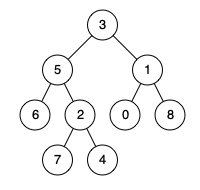# 236. lowest common ancestor of a binary tree

https://leetcode.com/problems/lowest-common-ancestor-of-a-binary-tree/

Given a binary tree, find the lowest common ancestor (LCA) of two given nodes in the tree. According to the definition of LCA on Wikipedia: “The lowest common ancestor is defined between two nodes p and q as the lowest node in T that has both p and q as descendants (where we allow a node to be a descendant of itself).”

Example 1:Input: root = [3,5,1,6,2,0,8,null,null,7,4], p = 5, q = 1 Output: 3 Explanation: The LCA of nodes 5 and 1 is 3. Example 2:Input: root = [3,5,1,6,2,0,8,null,null,7,4], p = 5, q = 4 Output: 5 Explanation: The LCA of nodes 5 and 4 is 5, since a node can be a descendant of itself according to the LCA definition. Example 3: Input: root = [1,2], p = 1, q = 2 Output: 1

Constraints:

``````The number of nodes in the tree is in the range [2, 105].
-109 <= Node.val <= 109
All Node.val are **unique**.
p != q
p and q will exist in the tree.
``````

• code
``````class Solution {
public TreeNode lowestCommonAncestor(TreeNode root, TreeNode p, TreeNode q) {
if (root.val == p.val || root.val == q.val || root == null) return root;
TreeNode left = null;
TreeNode right = null;
if (root.left != null) left = lowestCommonAncestor(root.left, p, q);
if (root.right != null) right = lowestCommonAncestor(root.right, p, q);
if (left != null && right != null) return root;
return left != null ? left : right;
}
}
``````
• code
``````class Solution:
def lowestCommonAncestor(self, root, p, q):
if root in (None, p, q): return root
left, right = (self.lowestCommonAncestor(kid, p, q)
for kid in (root.left, root.right))
return root if left and right else left or right

``````

It’s recursive and expands the meaning of the function. If the current (sub)tree contains both p and q, then the function result is their LCA. If only one of them is in that subtree, then the result is that one of them. If neither are in that subtree, the result is null/None/.